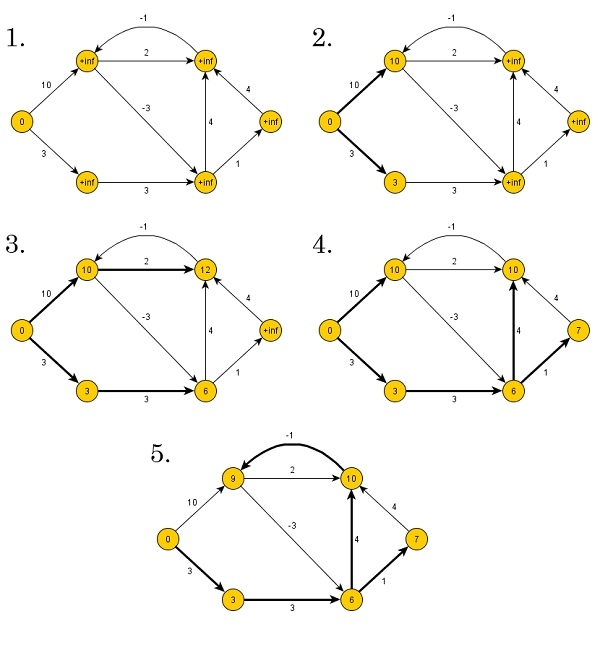# Single-Source Shortest Paths, Arbitrary Weights

The single source shortest path algorithm (for arbitrary weight positive or negative) is also known Bellman-Ford algorithm is used to find minimum distance from source vertex to any other vertex. The main difference between this algorithm with Dijkstra’s algorithm is, in Dijkstra’s algorithm we cannot handle the negative weight, but here we can handle it easily.Bellman-Ford algorithm finds the distance in bottom up manner. At first it finds those distances which have only one edge in the path. After that increase the path length to find all possible solutions.

Input − The cost matrix of the graph:

0 6 ∞ 7 ∞
∞ 0 5 8 -4
∞ -2 0 ∞ ∞
∞ ∞ -3 0 9
2 ∞ 7 ∞ 0

Output − Source Vertex: 2
Vert: 0 1 2 3 4
Dist: -4 -2 0 3 -6
Pred: 4 2 -1 0 1
The graph has no negative edge cycle

## Algorithm

### bellmanFord(dist, pred, source)

Input − Distance list, predecessor list and the source vertex.

Output − True, when a negative cycle is found.

Begin
iCount := 1
maxEdge := n * (n - 1) / 2 //n is number of vertices
for all vertices v of the graph, do
dist[v] := ∞
pred[v] := ϕ
done
dist[source] := 0
eCount := number of edges present in the graph
create edge list named edgeList
while iCount < n, do
for i := 0 to eCount, do
if dist[edgeList[i].v] > dist[edgeList[i].u] + (cost[u,v] for edge i)
dist[edgeList[i].v] > dist[edgeList[i].u] + (cost[u,v] for edge i)
pred[edgeList[i].v] := edgeList[i].u
done
done
iCount := iCount + 1
for all vertices i in the graph, do
if dist[edgeList[i].v] > dist[edgeList[i].u] + (cost[u,v] for edge i), then
return true
done
return false
End

## Example(C++)

#include<iostream>
#include<iomanip>
#define V 5
#define INF 999
using namespace std;
//Cost matrix of the graph (directed) vertex 5
int costMat[V][V] = {
{0, 6, INF, 7, INF},
{INF, 0, 5, 8, -4},
{INF, -2, 0, INF, INF},
{INF, INF, -3, 0, 9},
{2, INF, 7, INF, 0}
};
typedef struct{
int u, v, cost;
}edge;
int isDiagraph(){
//check the graph is directed graph or not
int i, j;
for(i = 0; i<V; i++){
for(j = 0; j<V; j++){
if(costMat[i][j] != costMat[j][i]){
return 1;//graph is directed
}
}
}
return 0;//graph is undirected
}
int makeEdgeList(edge *eList){
//create edgelist from the edges of graph
int count = -1;
if(isDiagraph()){
for(int i = 0; i<V; i++){
for(int j = 0; j<V; j++){
if(costMat[i][j] != 0 && costMat[i][j] != INF){
count++;//edge find when graph is directed
eList[count].u = i; eList[count].v = j;
eList[count].cost = costMat[i][j];
}
}
}
}else{
for(int i = 0; i<V; i++){
for(int j = 0; j<i; j++){
if(costMat[i][j] != INF){
count++;//edge find when graph is undirected
eList[count].u = i; eList[count].v = j;
eList[count].cost = costMat[i][j];
}
}
}
}
return count+1;
}
int bellmanFord(int *dist, int *pred,int src){
int icount = 1, ecount, max = V*(V-1)/2;
edge edgeList[max];
for(int i = 0; i<V; i++){
dist[i] = INF;//initialize with infinity
pred[i] = -1;//no predecessor found.
}
dist[src] = 0;//for starting vertex, distance is 0
ecount = makeEdgeList(edgeList); //edgeList formation
while(icount < V){ //number of iteration is (Vertex - 1)
for(int i = 0; i<ecount; i++){
if(dist[edgeList[i].v] > dist[edgeList[i].u] + costMat[edgeList[i].u][edgeList[i].v]){
//relax edge and set predecessor
dist[edgeList[i].v] = dist[edgeList[i].u] + costMat[edgeList[i].u][edgeList[i].v];
pred[edgeList[i].v] = edgeList[i].u;
}
}
icount++;
}
//test for negative cycle
for(int i = 0; i<ecount; i++){
if(dist[edgeList[i].v] > dist[edgeList[i].u] + costMat[edgeList[i].u][edgeList[i].v]){
return 1;//indicates the graph has negative cycle
}
}
return 0;//no negative cycle
}
void display(int *dist, int *pred){
cout << "Vert: ";
for(int i = 0; i<V; i++)
cout <<setw(3) << i << " ";
cout << endl;
cout << "Dist: ";
for(int i = 0; i<V; i++)
cout << setw(3) << dist[i] << " ";
cout << endl;
cout << "Pred: ";
for(int i = 0; i<V; i++)
cout << setw(3) << pred[i] << " ";
cout << endl;
}
int main(){
int dist[V], pred[V], source, report;
source = 2;
report = bellmanFord(dist, pred, source);
cout << "Source Vertex: " << source<<endl;
display(dist, pred);
if(report)
cout << "The graph has a negative edge cycle" << endl;
else
cout << "The graph has no negative edge cycle" << endl;
}

## Output

Source Vertex: 2
Vert: 0 1 2 3 4
Dist: -4 -2 0 3 -6
Pred: 4 2 -1 0 1
The graph has no negative edge cycle